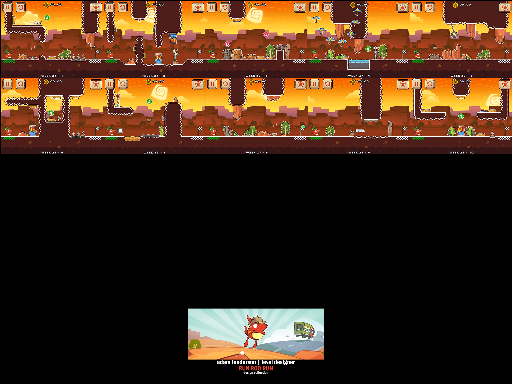role: LEVEL DESIGNER
As Level Designer, I designed and built all 690 levels that are available for Run Roo Run. Each chapter consisted of 15 Normal levels which introduced a new mechanic and allowed the player to get used to how it worked and how it interacted with any objects introduced in prior chapters. Along with the 15 Normal levels there were an additional 6 Extreme levels for each chapter that required near split second timing in some cases to be completed under par. Post launch, 10 new levels were uploaded weekly for 27 straight weeks bumping the total levels created for Run Roo Run to 690.

Launch Maps | Weekly Maps

levels: NORMAL/EXTREME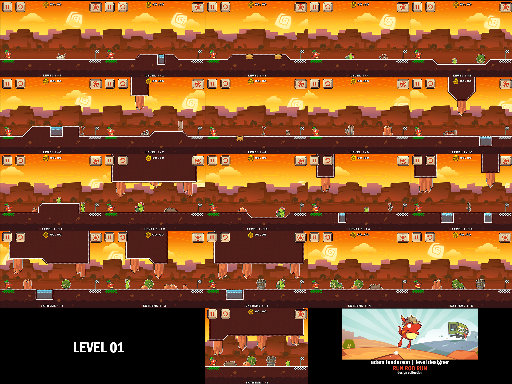<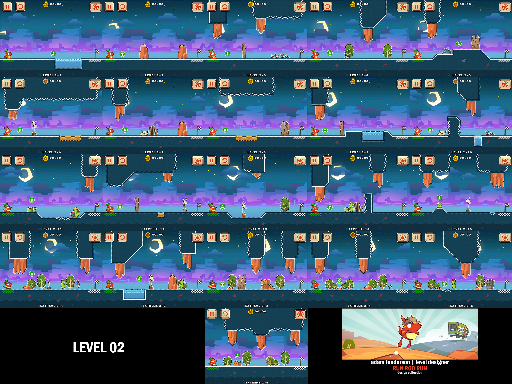<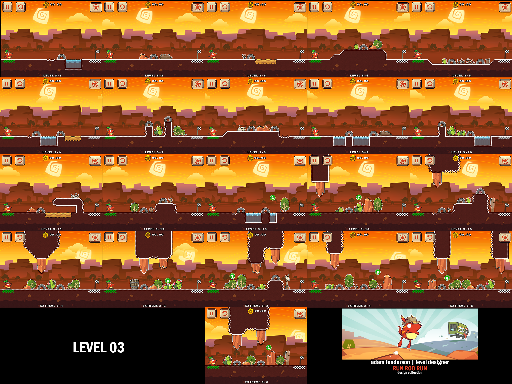<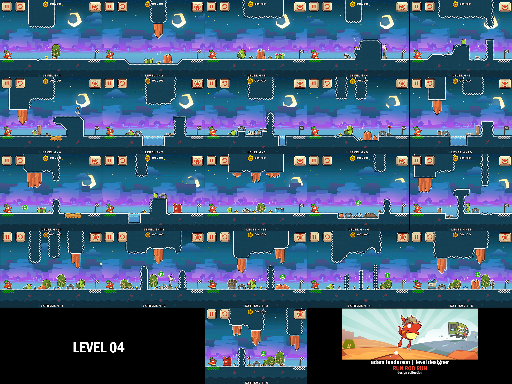<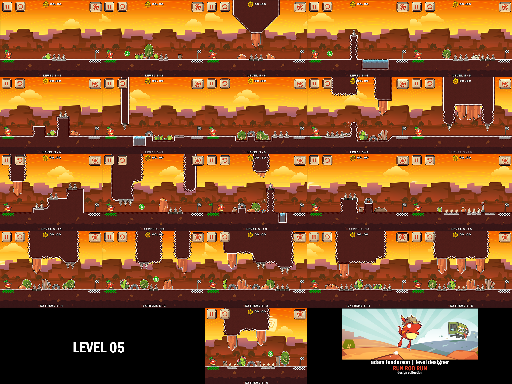<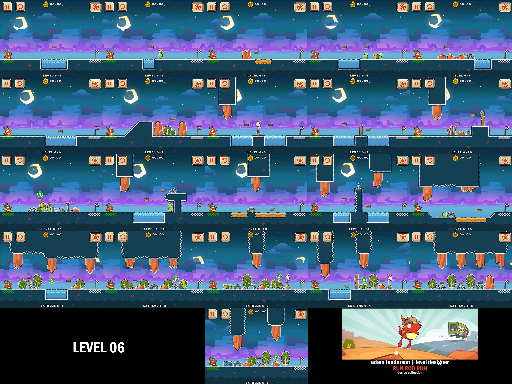<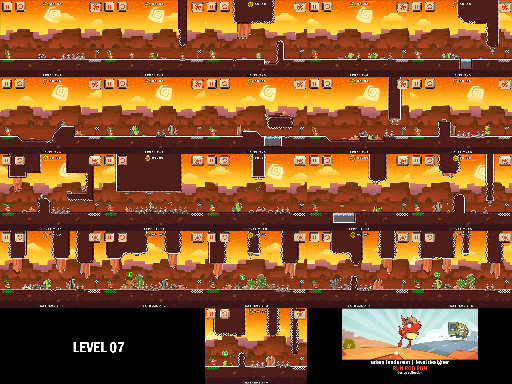<<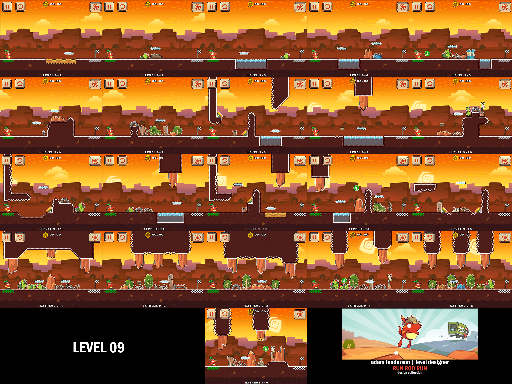<<<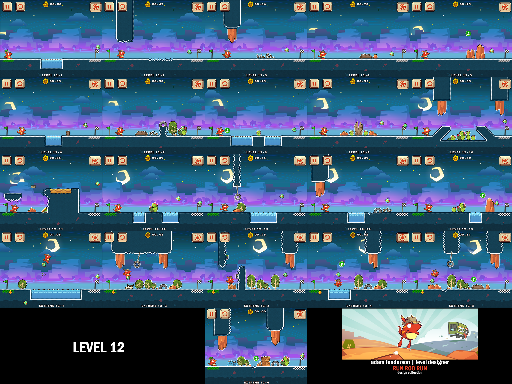<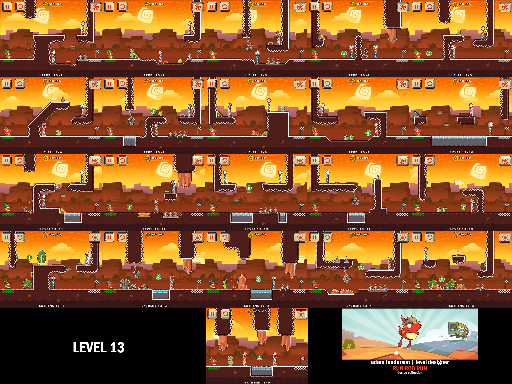<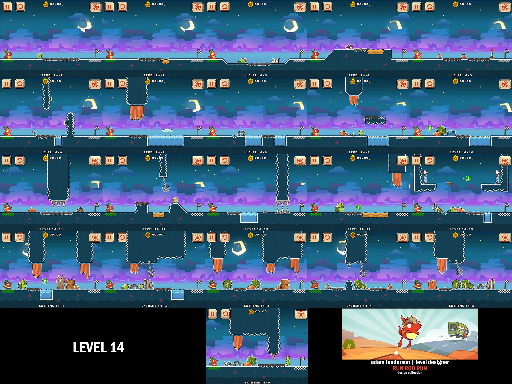<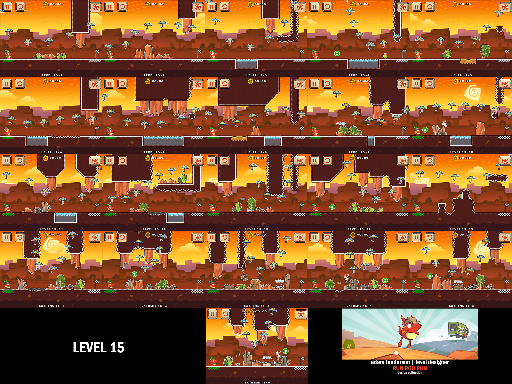<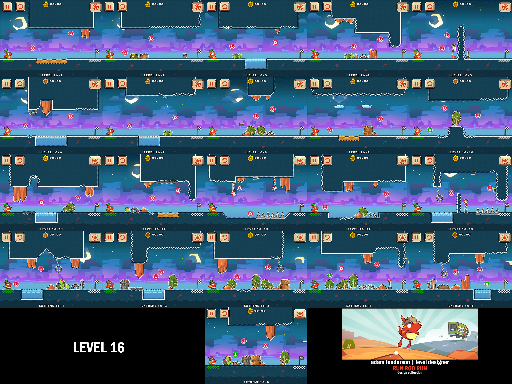<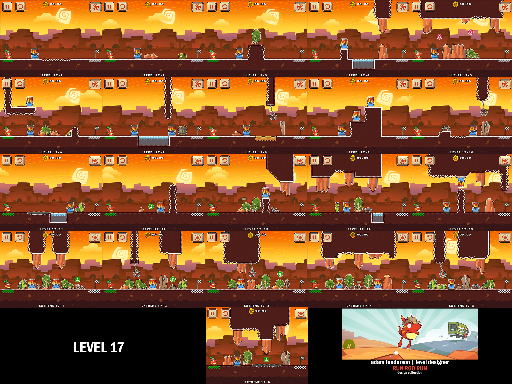<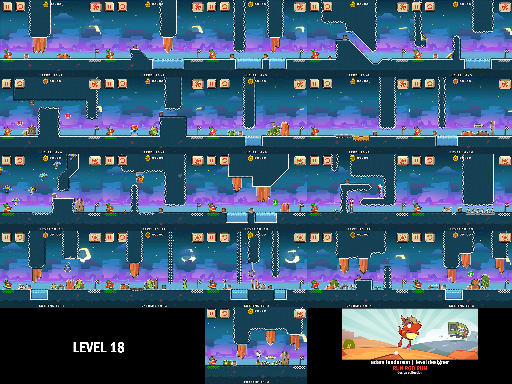<<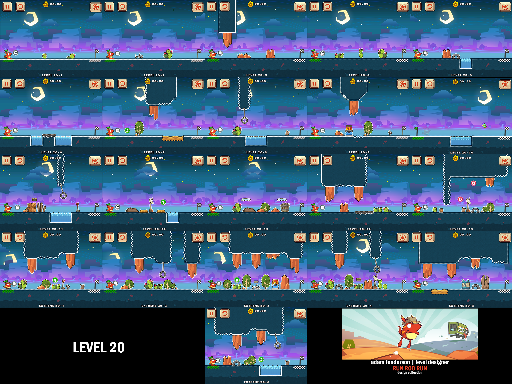<
levels: WEEKLY<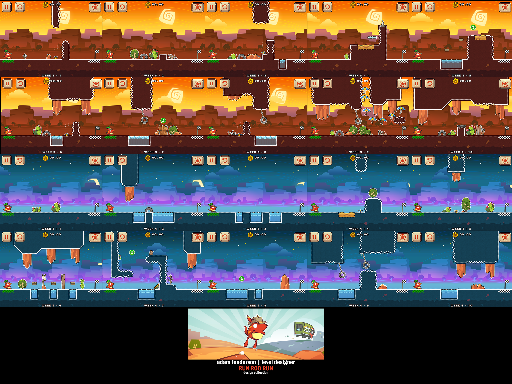<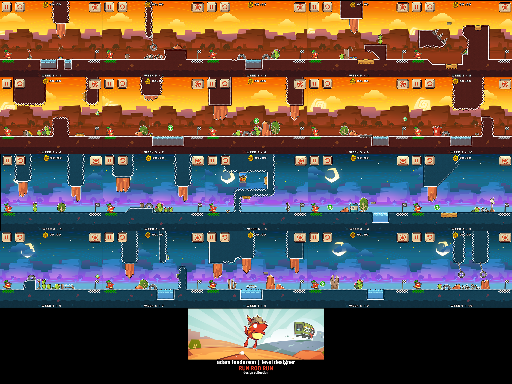<<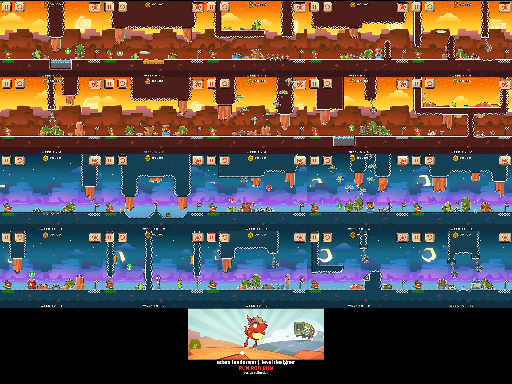<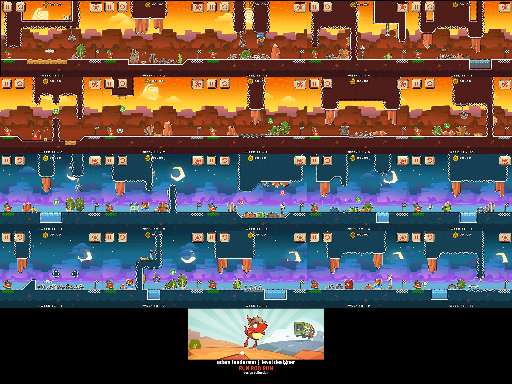<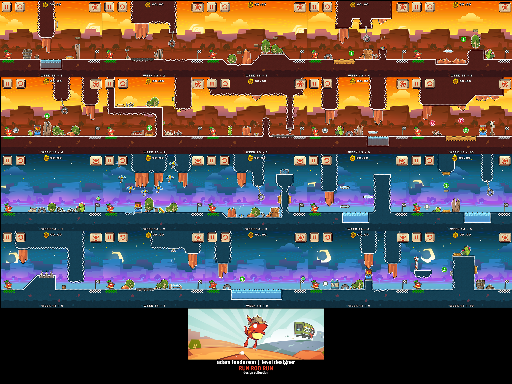<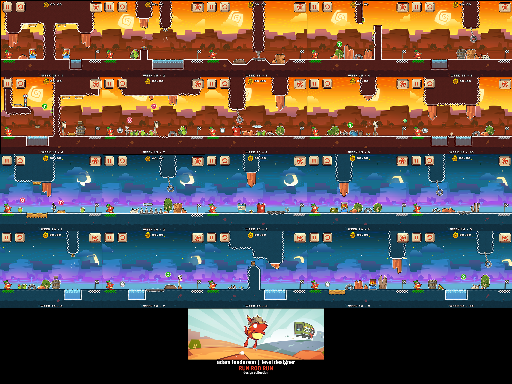<<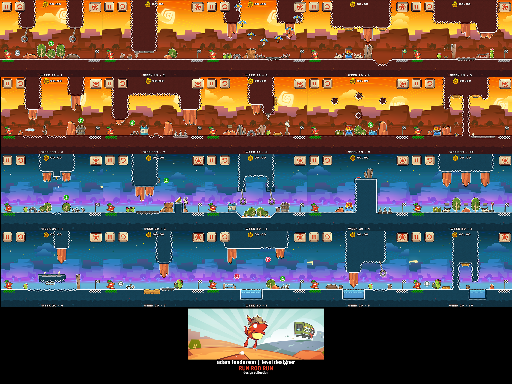<<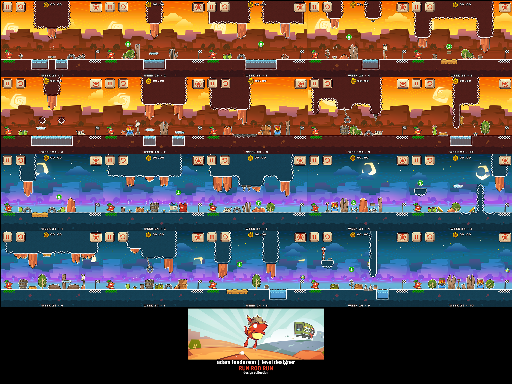<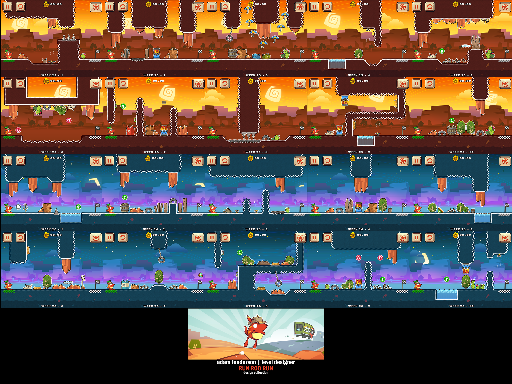<